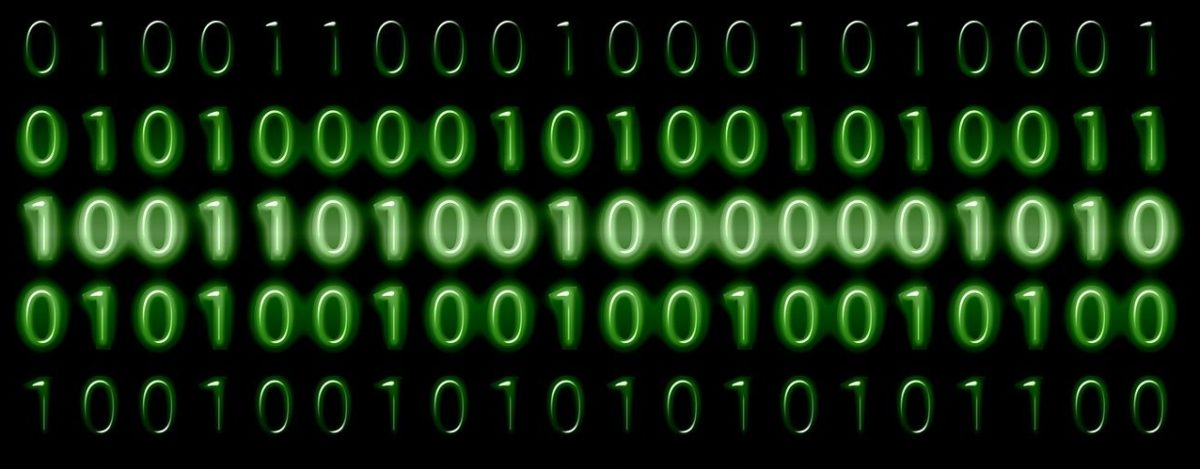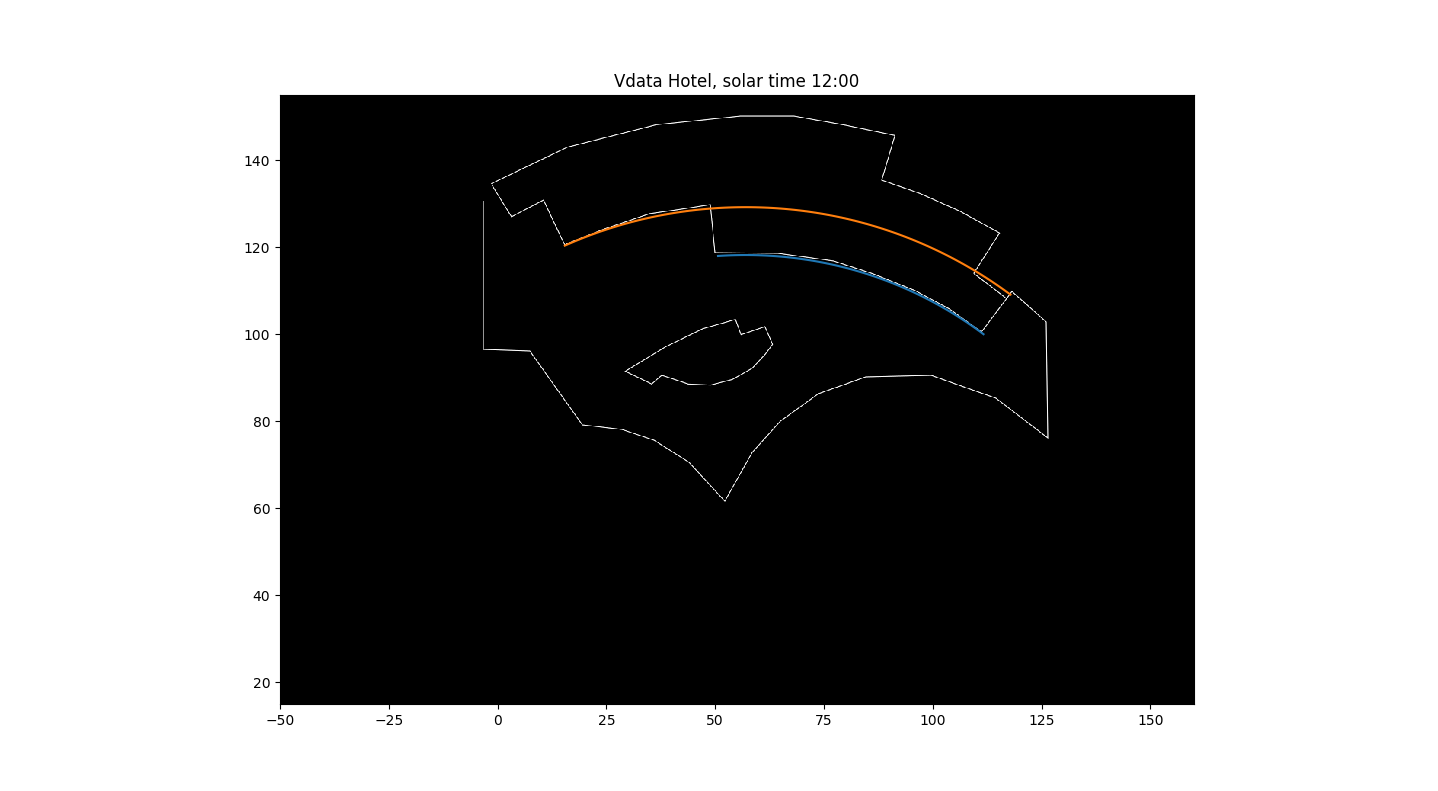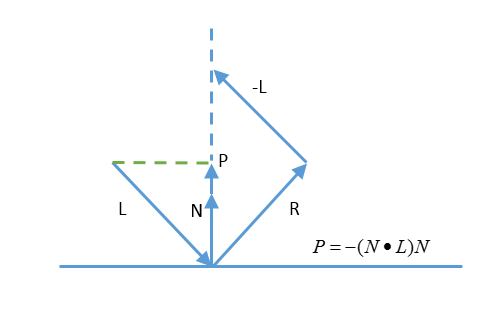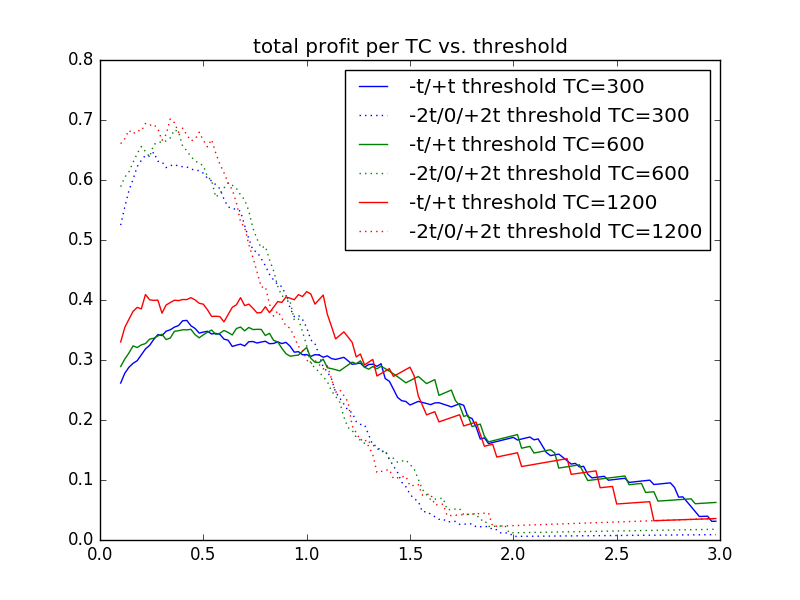# Bitwise Compression: What Is It and How Does it Work?

File compression programs such as Zip, Pkzip, and Winzip can make a file smaller and take up less space on your hard disk. These programs can then reverse the process, or uncompress the file(s), and restore them exactly as they were before. This is called lossless compression. There lossy compression programs, in which the restored files are close but not exactly the same as the original files. This might seem not useful for data files (like databases, text, or spreadsheets), but can be critically important for things like movies or photographic images. We are going to be only discussing lossless compression today.

The very best (in terms of compression ratio) programs use advanced techniques. For many more details and a history of progress, see Matt Mahoney’s Data Compression Explained. Matt Mahoney also runs the The Hutter Prize, a contest (with cash prizes) for the best programs in a specific data compression task. For reference, the current record for compressing the first 100MB of Wikipedia content has a compression ratio of 0.15285.

There is a specific technique that all the top compression programs use that I will explain in this blog post, called Bitwise Compression. We will get to that in a bit, but first we will need to discuss some basic data compression and information theory.

# Basic Compression

The basic idea of data compression is that repeated things can be assigned shorter codes than the length they originally were. This means that things that are more likely should be assigned shorter codes. Morse Code tries to be efficient by assigning the most common letter, “E”, the shortest code 1. Let’s look at a toy example, and suppose that we have just 4 symbols in a file and we want to compress the file. Suppose that the 4 symbols are A,B,C, and D and have the following probabilities:

SYMBOLPROBABILITY
A0.75
B0.15
C0.05
D0.05

The probabilities can either be thought of as the likelihood of those symbols appearing in future files that we will see, or the fraction of the symbols in a particular file that we are trying to compress. Note that in endeavors like the Hutter Prize, there is a specific file that we are trying to compress and optimize for that file as much as we want; general compression programs do not have that luxury.

Given the above symbols and probabilities, we might ask what is the optimal way to encode them in bits. A particular solution to this program was invented by David A. Huffman, in his 1952 paper, called Huffman Coding. He asked the question: if I assigned bit sequences to each of those symbols, what assignment would produce the shortest output bit sequence on average. Think of this like Morse Code, where instead of each symbol being assigned a certain sequence of dots and dashes, we assign a certain sequence of 0s and 1s.

For example, suppose we just decided to assign A=00, B=01, C=10, and D=11. That is, 2 bits for each symbol. Of course, this encoding method can be reversed, because we just take each pair of bits, and translate back into ABCD as we go. What is the average number of bits used per symbol for this code? By inspection, the answer is 2.0 bits per symbol. Dr. Huffman says we can do better. Using his algorithm, we get the following optimal assignment 2

SYMBOLCODELENGTHPROBABILITYCONTRIBUTION
A110.750.75
B0120.150.30
C00130.050.15
D00030.050.15
TOTAL1.35 bits/symbol

This assignment shows that 1.35 bits/symbol is achieved. Huffman says that is this the best we can do by assigning a bit sequence to each symbol. Are there other methods that could do better? Claude Shannon, who invented the entire field of Information Theory with his 2 part blockbuster paper published in the Bell System Technical Journal in 1948, said yes. He said that the information content of a symbol stream could be measured by somethings called Entropy, in units of bits. The formula for computing Entropy is

$E=\sum_{k=0}^{N-1}-p_i \log_2 p_i$

where $$\log_2$$ is the logarithm to the base 2, which, in case you have forgotten can be calculated as
\begin{align}
\log_2 x = \frac{\log x}{\log 2}
\end{align}
where log is the logarithm to your favorite base (such as the more common 10 or e). The units of Entropy are bits/symbol. The number of bits used for any entire file would then be Entropy times the number of symbols in the file.

For our toy ABCD example, the entropy we get is
$E=1.15402 \textrm{ bits/symbol}$

Now, Shannon did not know the exact practical way to get to an encoding method that could achieve this, but he showed that it was possible. One way to do better would be to encode pairs of symbols into Huffman Codes. That is, we make a table like we did above, but the symbols are now AA, AB, AC, AD, BA, etc. We get the probabilities of these combinations by multiplying the probabilities of the individual symbols together (putting aside conditional probabilities for the time being). This will get us closer to the 1.15402 bits/symbol we are looking for, but not there yet. The reason is that we constrained to using a whole number of bits for each symbol and we end up wasting output bits for each symbol.

# Arithmetic Coding

Enter Arithmetic Coding, invented in the 1970s at IBM, and published in the IBM Journal of Research and Development. It basically allows us to out fractional bits for each symbol, and overall, the number of bits used approaches the Entropy we calculated above (the Arithmetic Coding algorithm gets us to within 1 byte of the Entropy in our entire sequence, so let’s call that good enough). There are many descriptions on how AC works, starting with Arithmetic Coding. We are not going to go into the details here.

# Context

In any real file, the individual bytes do not show up with independent probabilities. For example, in an English language text file, after the letter “q”, the letter “u” is very likely to show up, far more likely than it’s overall frequency of occurrence in the file. How do we take advantage of this information? It turns out, that using Arithmetic Coding (AC), it very straightforward. AC, at each step, just needs the probabilities of each symbol, and it can output the proper bit sequence to encode those symbols. The decoder just needs the same probabilities provided to it, and it can recover the original symbol sequence. One way that file compression algorithms can take advantage of this information is by building models (or tables) as a file is processed, which try to predict what the next symbol will be. This prediction is done by giving a probability for each symbol. Now, in a technique known as Prediction by Partial Matching (PPM), we look at the previously seen symbols and then predict the next symbol. For example, if we see “sequ” we might give a high probability to the next symbol being “e”. This is done by keeping track of how many times various symbols are seen for each context, or previous symbols. Where it gets difficult is that you want the probabilities to be adaptive, so they can adjust to varying parts of the file. It turns out that we cannot just compute these probabilities by context for the entire file and use those for encoding. They would have to sent along to the decoder. While this would result in extremely good compression ratios, the size of the data (the probability for every context) would be huge, and negate the space savings.

For a bunch of reasons, a variation of this method, Bitwise Compression, was developed. It works basically the same way, but each symbol being encode is a bit from the source file. That is, we only have 2 symbols, “0” and “1”. This makes everything simpler, including the AC algorithm, which now only needs the probability of a 1 to encode each bit. A good question to ask is, are we losing anything by doing this Bitwise Compression? At first glance, it seems like you might be taking up extra space because each bit has to be encoded, rather than entire bytes.

# Bitwise Compression

Now, one thing is obvious. If we did not use any context, this would not work. Without any context, each bit is likely to be 50/50, so we would not gain any compression benefit. So what I want to show here is that compressing by bytes is equivalent to compressing by bits, with the context of the byte encoded so far.

Let’s start by comparing two methods of compressing a text file:
(1) Compress each byte based on its probability of occurrence in the file, with no context. This is easily estimated by simply computing the probability of each byte (based on the number of times it occurs in the file), then computing the entropy of those probabilities.
(2) Compress each byte, one bit at a time, where the context of each bit prediction is the previously seen byte. That is, for the first bit prediction, the context is nothing. That is, the first bit will be predicted based on the probability of the first bit of each byte being 1. The second bit will be predicted based on the previous context being ‘0’ or ‘1’, depending on what the first bit was. A simple way to handle the bit context in Python is to make a context string, which is a ‘1’ followed by the actual bits seen so far. This distinguishes 3 zeros seen from 1 zeros seen: 1000 (0x8) means 3 zeros seen, while 10 (0x3) means a single 0 seen, and 1 (0x1) means nothing seen yet (the first bit of a byte). In the accompanying code, this is called the bitcontext.

You can follow along with the python code in the related gitlab account, which you can get by doing

git clone https://gitlab.com/dgrunberg/bitwise-compress.git

If you have Python 3.5 or later, you should be able to run this code, like this:

python study-bits.py --meg 1 --input enwik8

Where enwik8 is the 100MB of Wikipedia data you can get from the Hutter Prize site. This is perform the analysis on the first 1000000 bytes of enwik8. The Estimated compression bits it puts out is computed by calculating the entropy of the probabilities generated as inputs to the AC.

The results are

Method – no previous bytes context + bitcontext Compression Ratio
Entropy by byte: 5.0589 bits/byte 0.6324
Encode by bit – Estimated bits out by calculating entropy 0.6324
Encode by bit – Actual bits output 0.6324

So you can see, for this simple example file, that encoding by bit with a single byte bitcontext gives the same result as encoding based on byte probabilities.
Now, for a preview of how context can help with encoding, let us consider using the single previous byte as context. The results are:

Method – single previous byte as context + bitcontext Compression Ratio
Encode by bit – Estimated bits out by calculating entropy 0.4793
Encode by bit – Actual bits output 0.4794

So we went from compression ratio of 0.6324 to 0.4794 by using a single previous byte as context. If you follow the history of compression programs from Matt Mahoney’s excellent site, you will see that to get from here to the current record holder ratio of about 0.15, a number of additional things get added:

1. An adaptive probability model is used, where the count of occurrences of each context is encoded into a single byte (called a bit history). This gets updated each time a context is encountered through a table lookup
2. Each count is mapped to a probability through a single table for the entire context; the table gets updated as each bit is revealed.
3. Many different models are used, including various numbers of previous bytes and some unigram word models
4. These models are combined linearly (called the mixer), with the weights adjusted adaptively
5. This mixed probability is then run through a SSE (Secondary Symbol Estimation), which is another adaptively updated table that corrects this probability. Sometimes 2 or more SSE stages are used.
6. More than 1 set of weights are used, where the particular weight to be used is chosen based on some other context characteristics.
7. A preprocessor and dictionary is used, which replaces many of the words in the file with various dictionary entries and escape codes.

-THE END

Notes:

1. E is just one single dot. The second most common letter, T, is a single dash.
2. One minor point I glossed over: since the codes in general are different lengths, we have to be able to decode them without any specific indication of where each code ends. That is, we need to be able to parse out the individual bit sequences from the overall stream. The property of the chosen codes that ensures this will happen is called the prefix property. This means that no code can be a prefix (first part) of any other code. Huffman Codes have this property.# Starting a Fire With a Hotel

The Vdara hotel in Las Vegas gained some notoriety in 2010 when guests complained about getting severe burns from the pool deck area (see article). Note the curved facade and the pool and deck immediately below.  Image By Cygnusloop99 (Own work) [CC BY-SA 3.0 or GFDL], via Wikimedia Commons

Here is the view from Google maps. We will use this to get some measurements for the hotel:

We are going to build a simple simulation of the solar radiation reflection off the hotel mirrored facade. To this, we will need some things:

1. The geometry of the hotel and pool deck area
2. The orientation of the sun by time and date
3. Calculations for reflection of the sun’s rays and their intersection with the pool deck.

Let’s take them in order.

# Hotel Geometry

From a hotel floor plan, easily obtained on the web, we trace out the pool, pool deck, and facade surfaces.  Using the above satellite photo to get the scale properly, we then create 2 circular surfaces that closely match the facade geometry.  The radii of the 2 facades are 91.2m and 112.2m.  This is what the mathematical curves overlaid on the image look like. The axes are in meters and North is facing upward.  Notice that the curved surfaces have a very nice southern exposure.In this image, I have extended the second wall (orange) all the way in order to see how it fits on the floor plan. It is slightly above the floor plan at its right-most edge because there is a slight separation between the 3 semi-circular facades.
You can see the original floor plan here (rotated) Vdara Floor Plan.

# Sun Orientation

The Wikipedia Sun Position article has a good introduction to the basic calculations.  We will review what we need here.  Basically, there are two coordinate systems that we will need.  The first is the ecliptic coordinate system, in which the sun and stars are (roughly speaking) constant.  Think of it as the stars’ coordinate system. In it, the ecliptic latitude is the angle from the plane of the Earth’s orbit around the Sun.  The ecliptic longitude of the Sun is zero when the Earth is at the vernal equinox.  So the ecliptic latitude of the Sun is 0, and the longitude depends on the day of the year, at least to the precision that we need for our solar convergence calculations.

The other coordinate system that we will need is the horizontal coordinate system.  This coordinate system is referenced to a viewer on a spot on the Earth, and has

• Solar altitude: the angle above the horizon.  Its complementary angle is the Solar zenith.
• Solar azimuth: the angle from the North, increasing clockwise towards the East.  Thus, 90 degrees is East and 180 degrees is South.

Rather than give all the equations for the calculations, which you can find on the Wikipedia page, I will give a Python function that will calculate the Sun’s position. The hour is the time before/after Solar Noon, which is when the Sun is at it’s highest point in the sky (close to Noon local time), and simpler to use here than trying to calculate from local time (which requires knowledge of time zones, etc.). Since we will be computing the Sun’s angle as it moves through the sky, the exact time is not important to us. If you want to check out when solar noon occurs, and compare the function below for accuracy, see NOAA Solar Calculations. For Las Vegas, the NOAA site matched this function within a few tenth’s of a degree.

def sun(hour, dt_noon=None):
#compute sun at hour before/after solar noon on dt_noon, e.g. hour=-1 is 1 hour before solar noon
if dt_noon is None:
dt_noon=datetime.datetime(2017,8,24,hour=12,minute=0,second=0)
#ecliptic coordinates
n=(dt_noon - datetime.datetime(2000,1,1,hour=12)).days  #number of days since 1/1/2000
L=(280.46+.9856474*n)/180*math.pi
g=(357.528+.9856*n)/180*math.pi
L=angle(L)  # make sure in 0-2*pi range
g=angle(g)
ecliptic_longitude=L+(1.915*math.sin(g)+0.020*math.sin(2*g))/180*math.pi
ecliptic_latitude=0.0
obliquity_of_ecliptic=23.4/180*math.pi

hour_angle=(hour*15)/180*math.pi
sun_declination=math.asin(math.sin(obliquity_of_ecliptic) * math.sin(ecliptic_longitude))

solar_altitude=math.asin(math.sin(local_latitude)*math.sin(sun_declination)+
math.cos(local_latitude)*math.cos(sun_declination)*math.cos(hour_angle))
solar_zenith=math.pi/2-solar_altitude
#solar_azimuth=math.pi-math.asin(-math.sin(hour_angle)*math.cos(sun_declination)/math.sin(solar_zenith))
#solar_azimuth is measured by 0=north, 90 degrees=East, 180=South.
#Note: problem when asin argument is around 1, because there are 2 solutions (like 85 and 95), so
#we might get the wrong answer around sunrise/sunset.
#This alternate version does not have the same problem, but we have to be careful about sign of h
n1=math.sin(sun_declination)*math.cos(local_latitude) -
math.cos(hour_angle)*math.cos(sun_declination)*math.sin(local_latitude)
d1=math.sin(solar_zenith)
if (n1/d1) >= 1.0:
solar_azimuth=0
elif (n1/d1<-1.0):
solar_azimuth=math.pi
elif h<0:
solar_azimuth=math.acos(n1/d1)
else:
solar_azimuth=2*math.pi-math.acos(n1/d1)
return solar_altitude, solar_azimuth



# Reflections and Intersections

The last piece we need are the calculations for reflections off surfaces.  If a light ray vector L impinges on a surface with unit normal N, it will be reflected with the same angle opposite the normal.  Looking at this figure:We can see that
\begin{align}
P=-(N \cdot L)N
\end{align}

because it is the projection of L onto the normal N.  Then we have

\begin{align}
R-L&=2P \\
R&=L-2(N \cdot L)N
\end{align}

Now that we have the reflected ray, we need to see how it intersects with the ground (or pool deck). For a plane described by a point $$p_0$$ and normal $$n$$ containing all points p such that:
\begin{align}
(p-p_0) \cdot n = 0
\end{align}

and a line described by a point $$l_0$$ and a vector in the direction of the line $$l$$ where the line is
\begin{align}
p=dl+l_0
\end{align}
points p for all real numbers l. If we solve these 2 equations for d, we get:
\begin{align}
d=\frac{(p_0-l_0) \cdot n}{l \cdot n}
\end{align}
which we can substitute back into the previous equation to get the intersection point $$p$$.

# Putting It All Together

Using Python and matplotlib, we can easily piece together a simulation of the intensity of the Sun’s rays.  To start, I tested the program with a simple flat facade in roughly the orientation of the first Vdara curved wall.

The red line, at approximately -18 degrees, is the mirrors position on the floor plan.  The wall is 176m high, which is the height of the Vdara hotel.

The solar intensity is about 1.0 for the entire parallelogram image, which sort of what we would expect for a flat mirror.  It is not exactly 1 because the sun is not hitting the pool deck at exactly 90 degrees.  The 3 yellow lines represent the Sun’s azimuth.  Because this is solar 12:00 (solar noon), the azimuth is exactly 180 degrees.

Now, let us look at the results for the curved facade.

Wow.  You can things are starting to heat up.  Believe it or not, the peak solar value is 15 in this plot.  A few things to be careful about:

• I have not modeled the different heights of the 3 curved walls, nor the third wall that extends a little bit beyond the second wall
• I have not modeled rays that have 2 or more reflections from their first intersection with the wall.  That is, some rays can hit the wall at an oblique enough angle to then hit the wall again.  In the figures to come, you can see that because some rays accumulate behind the wall, which would not happen in reality.
• I have assumed that the walls are 100% reflective with no loss.  The architect did place some kind of plastic covering to reduce the reflection; how much has not been reported.  Also, with glass windows, some of the light passes through the window; how much is transmitted vs. reflected depend on the angle of incidence and the index of refraction of the glass.  A more accurate model would take this into account.

Now, placing several of these figures in an animation around solar noon, we can see how the hot spots move across the pool deck:

If you haven’t read Part 1, do that first.
$$\newcommand{\Var}{\mathrm{Var}}$$
$$\newcommand{\Cov}{\mathrm{Cov}}$$
$$\newcommand{\Expect}{{\rm I\kern-.3em E}}$$
$$\newcommand{\me}{\mathrm{e}}$$
For a quick review, recall that our system looks like:
\begin{align}
x_{n+1}&=ax_n+w_n \\
w_n &\sim N(0, \sigma^2) \\
|a| & < 1. \\
\end{align}
Next, let’s define a time constant, $$\tau$$, that gives us an idea of how the noise term, $$w_n$$, is filtered. Time constants are generally the time it takes to reach $$1/\me$$ of a steady-state value, so:
\begin{align}
&\frac{1}{\me}=a^\tau \\
&-1=\tau \ln a\\
&\tau=\frac{-1}{\ln a}\\
\text{or}\\
&a=\me^{-1/ \tau}
\end{align}

Now, our goal is to understand how we could trade (profitably) such a stochastic system. What does this mean? We might want to try something like, “buy low, sell high.” Like
\begin{align}
x_n \geq T_2&\Rightarrow \text{sell}
\end{align}
Our profit on each round-trip trade would be $$T_2-T_1$$. Hopefully, we choose $$T_2 > T_1$$.

## Thresholds

Since our system is linear, scaling the input ($$w_n$$), scales the output. Thus, if we set thresholds as a multiple of $$\sigma$$ we do not have to explore different noise powers. Put another way, if we fix, say, $$\Var(x)\equiv 1$$, then we can experiment with different thresholds, and the results will hold for those thresholds as a multiple of $$\Var(x)$$.

## Time Durations

Similarly, if we are counting events (trades) over time, we want to be able to scale everything to a useful value.
Consider (from Part 1):

\begin{align}
\Var(x_k-x_0)&=2 \sigma^2 \frac{1-a^k}{1-a^2} \\
&=2\Var(x)(1-a^k)\\
&=2\Var(x)(1-\me^{-k/\tau})
\end{align}
We can see that the variance of increments depends on the number of time constants that we go out. So, if we count trades per time constant interval, we will have standardized our results. Note: The astute reader should note that this is not a proof (not even close), but we will leave that for later. This is more of a “seems plausible.”

## Some Simulations

Let’s perform a few tests to get started. We will pick an $$a$$ corresponding to $$\tau$$ of [300, 600, 1200] time steps (think seconds), and then fix $$\sigma$$ to make $$\Var(x)\equiv 1$$. Then we will perform Monte Carlo simulations over enough time steps to get statistically significant results.

There are 2 types of trading algorithms simulated here:

• -t/+t: buy when x<-t and sell when x>+t, count action as 0.5 trades
• -2t/0/+2t: buy when x<-2t and sell when x>0; plus sell when x>2t and buy when x<0

Each of these has a 2t profit per round trip trade, but the second option occurs more often for smaller thresholds.  For larger thresholds, the rapid Gaussian falloff causes there to be fewer trades.

Note you can see that the three different time constant (TC) values have lines very close to each other, justifying our supposition that events per TC are the relevant experimental parameter.  This means we can do our simulations for a single TC, rather than a whole series.

If we multiple the number of trades by the profit per trade, we get the following result.  Of course, this ignores commissions, which would drag down the profits more on the lower thresholds (more trades).The simulations used 200,000 time steps, and the following parameters.

time constant (steps)astd dev of wstd dev of x
300.99667.0821.031
600.99833.0581.029
1200.99917.0411.005